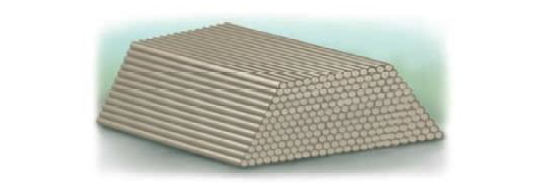Chapter 13.2, Problem 72E### Algebra and Trigonometry (MindTap ...

4th Edition
James Stewart + 2 others
ISBN: 9781305071742

#### Solutions

Chapter
Section### Algebra and Trigonometry (MindTap ...

4th Edition
James Stewart + 2 others
ISBN: 9781305071742
Textbook Problem

# Poles in a Pile Telephone poles are being stored in a pile with 25 poles in the first layer, 24 in the second, and so on. If there are 12 layers, how may telephone poles does the pile contain?To determine

To find:

Number of telephone poles in the pile

Explanation

Given:

Number of poles in first layer is 25

Total number of layers 12

Number of poles decreases by 1 as we move down the pile of poles.

Approach:

Show that the number of poles in consecutive layers forms an arithmetic sequence and find sum of this arithmetic sequence to find number of poles.

Calculation:

Number of poles decreases by d=1

Number of poles in first layer, a=25

Number of layers, n=12

So the number of poles in consecutive layers as move down the pile forms the sequence:

25,24,23,22,...

which is an arithmetic sequence with first term a and common difference d.

Hence the nth term is a(n1)d which gives the number of poles in nth layer

### Still sussing out bartleby?

Check out a sample textbook solution.

See a sample solution

#### The Solution to Your Study Problems

Bartleby provides explanations to thousands of textbook problems written by our experts, many with advanced degrees!

Get Started